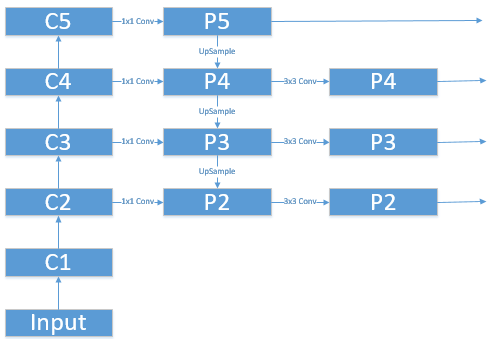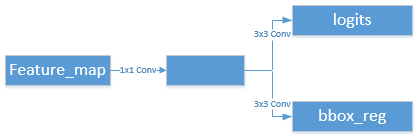# 深度学习笔记（十六）Faster RCNN + FPN (PyTorch)

08/10 07:19

Faster R-CNN: Towards Real-Time Object Detection with Region Proposal Networks

Feature Pyramid Networks for Object Detection

# 一. 总览

Faster RCNN 从功能模块来看，可大致分为 特征提取RPNRoI PoolingRCNN 四个模块，这里代码上选择了 ResNet50 + FPN 作为主干网络：

model = torchvision.models.detection.fasterrcnn_resnet50_fpn(pretrained=False)

## 1.1 特征提取# 二. RPN

## 2.1 Anchor Generator

以官方 PyTorch torchvision 里的 Faster RCNN 代码为例：输入图片尺度为 768x1344，5 个 feature map 分别经过了 stride=(4, 8, 16, 32, 64)，得到了 5 个大小为 （192x336, 96x168, 48x84, 24x42, 12x21) 的 feature。

[-23., -11., 23., 11.]    [-45., -23., 45., 23.]    [-91., -45., 91., 45.]
[-16., -16., 16., 16.]    [-32., -32., 32., 32.]   [-64., -64., 64., 64.]
[-11., -23., 11., 23.]    [-23., -45., 23., 45.]    [-45., -91., 45., 91.]

[-181., -91., 181., 91.]       [-362., -181., 362., 181.]
[-128., -128., 128., 128.]   [-256., -256., 256., 256.]
[ -91., -181., 91., 181.]      [-181., -362., 181., 362.]

(192*336*3=193536) + (96x168*3=48384) + (48*84*3=12096) + (24*42*3=3024) + (12*21*3=756) = 257796 个 Anchor

anchors = self.anchor_generator(images, features)有多少 Anchor 就有多少分类和回归结果，最终多个尺度（FPN 5 个尺度）cancat 后的分类维度为 257796x1, 回归维度为 257796x4。值得注意的是这里的回归结果是基于编码后的 Anchor 的偏移量。说道这里就要将下 Faster RCNN 里的 encode 和 decode 过程。区别于之前的 SSDYOLOV3 两个检测算法的编解码方式：

### 解码：

proposals = self.box_coder.decode(pred_bbox_deltas.detach(), anchors)

\begin{equation}
\label{decode}
\begin{split}
& x =  t_x * w_a + x_a \\
& y =  t_y * h_a + y_a \\
& w =  e^{t_w} * w_a \\
& h =  e^{t_h} * h_a \\
\end{split}
\end{equation}

### 编码：

regression_targets = self.box_coder.encode(matched_gt_boxes, anchors)

\begin{equation}
\label{encode}
\begin{split}
& t_x^* = (x^* - x_a) / w_a \\
& t_y^* = (y^* - y_a) / h_a \\
& t_w^* = log(\frac{w^*}{w_a}) \\
& t_h^* = log(\frac{h^*}{h_a}) \\
\end{split}
\end{equation}

## 2.3 筛选 Proposal

boxes, scores = self.filter_proposals(proposals, objectness, images.image_sizes, num_anchors_per_level)

# 三. RoI Pooling

有了筛选出来的 Proposal，我们就可以将映射到某个 feature map 上然后利用 RoI Pooling 提取每个 Proposal 的特征供后续的 rcnn 细致的分类和回归了。

box_features = self.box_roi_pool(features, proposals, image_shapes)

\begin{equation}
\label{level}
k = \lfloor k_0 + log2(\sqrt{wh}/224) \rfloor
\end{equation}

result_idx_in_level = roi_align(
per_level_feature, rois_per_level,
output_size=self.output_size,
spatial_scale=scale, sampling_ratio=self.sampling_ratio)

# 四. RCNN

self.cls_score = nn.Linear(in_channels, num_classes)
self.bbox_pred = nn.Linear(in_channels, num_classes * 4)

0
0 收藏

### 作者的其它热门文章0 评论
0 收藏
0